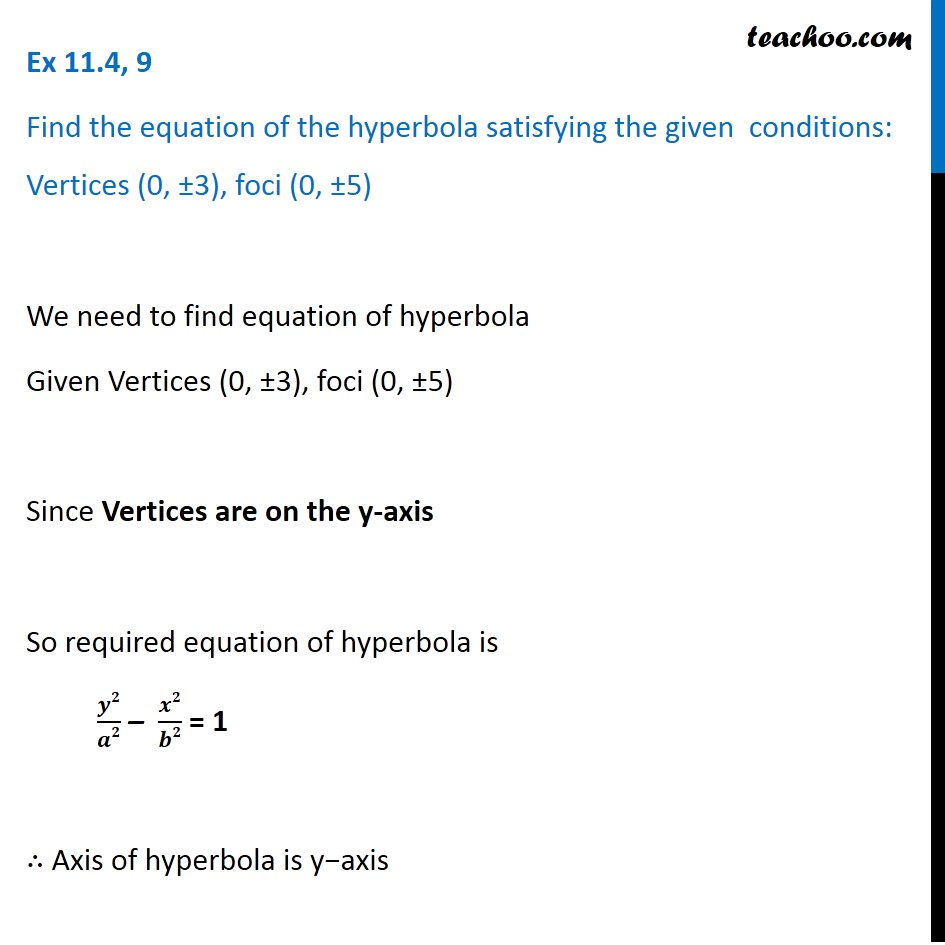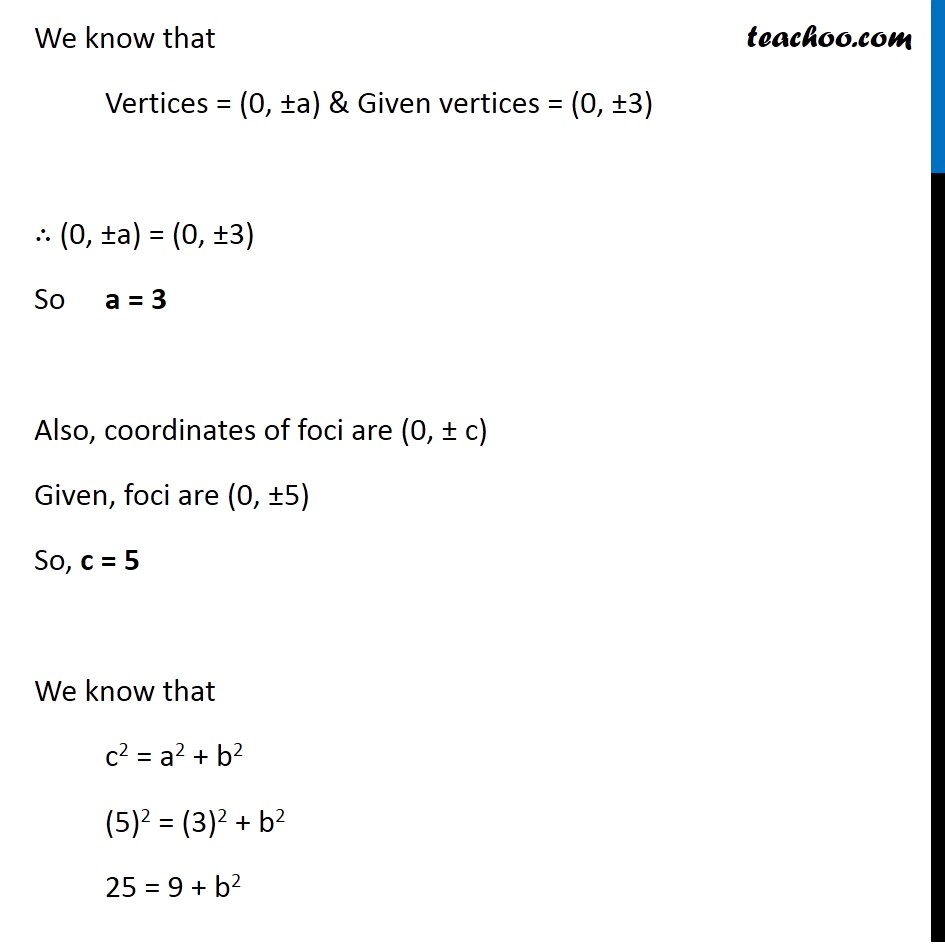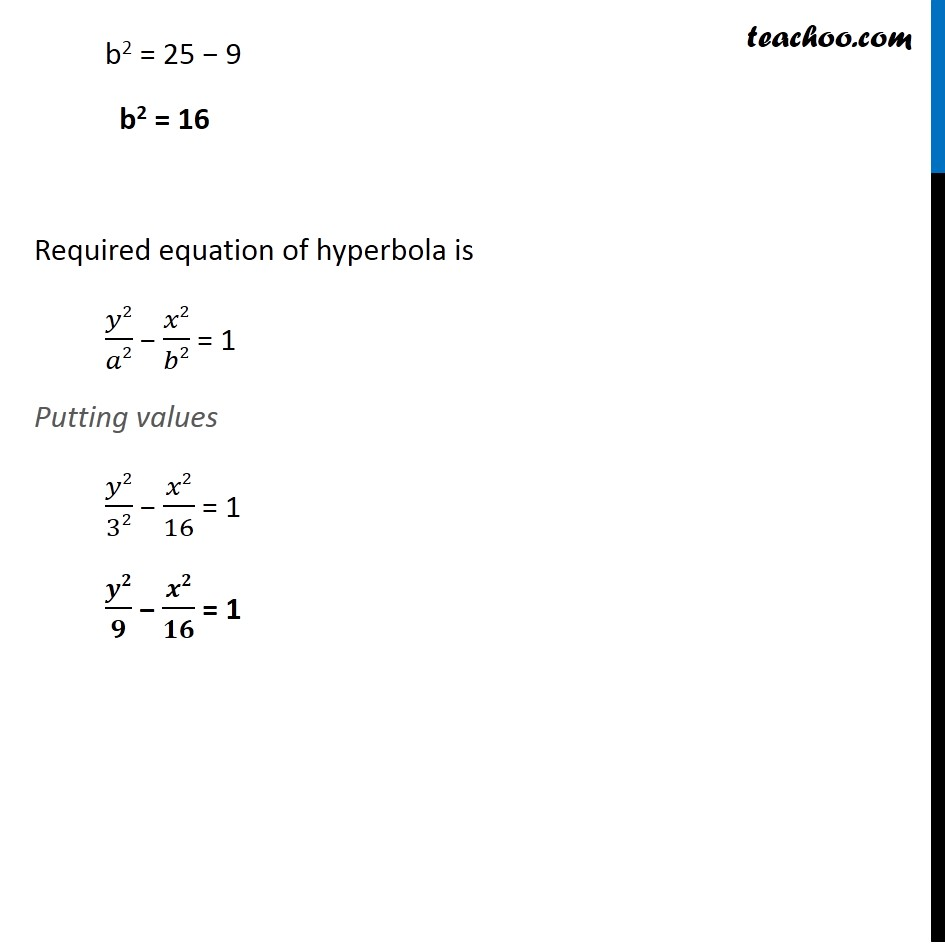1. Chapter 11 Class 11 Conic Sections (Term 2)
2. Serial order wise
3. Ex 11.4

Transcript

Ex 11.4, 9 Find the equation of the hyperbola satisfying the given conditions: Vertices (0, ±3), foci (0, ±5) We need to find equation of hyperbola Given Vertices (0, ±3), foci (0, ±5) Since Vertices are on the y-axis So required equation of hyperbola is 𝒚𝟐/𝒂𝟐 – 𝒙𝟐/𝒃𝟐 = 1 ∴ Axis of hyperbola is y−axis We know that Vertices = (0, ±a) & Given vertices = (0, ±3) ∴ (0, ±a) = (0, ±3) So a = 3 Also, coordinates of foci are (0, ± c) Given, foci are (0, ±5) So, c = 5 We know that c2 = a2 + b2 (5)2 = (3)2 + b2 25 = 9 + b2 b2 = 25 − 9 b2 = 16 Required equation of hyperbola is 𝑦2/𝑎2 − 𝑥2/𝑏2 = 1 Putting values 𝑦2/32 − 𝑥2/16 = 1 𝒚𝟐/𝟗 − 𝒙𝟐/𝟏𝟔 = 1

Ex 11.4

Chapter 11 Class 11 Conic Sections (Term 2)
Serial order wise